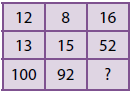# User Forum

Subject :Rea    Class : Class 4

If the given matrix follows a certain rule row-wise or column-wise, then find the missing number.

(NCO 2014)A272
B181
C77
D50

Solution pls

## Ans 1:

Class : Class 8
Answer is A. THIS WAS A REALLY NICE AND HARD QUESTION. Logic------Rule is column-wise that is vertically. Add the first two numbers and then multiply by four. Example- 12+13=25, 25*4=100 Same for- 8+15=23, 23*4=92 So- 16+52=68, 68*4=272

Class : Class 3
Thanks

## Ans 3:

Class : Class 4
thanks for the answer it was very difficult

Class : Class 2
thanks

Class : Class 6

Class : Class 4
ans is a

Class : Class 4

## Ans 8:

Class : Class 6
Thanks for the logic

## Ans 9:

Class : Class 5
Answer is A. THIS WAS A REALLY NICE AND HARD QUESTION. Logic------Rule is column-wise that is vertically. Add the first two numbers and then multiply by four. Example- 12 13=25, 25*4=100 Same for- 8 15=23, 23*4=92 So- 16 52=68, 68*4=272